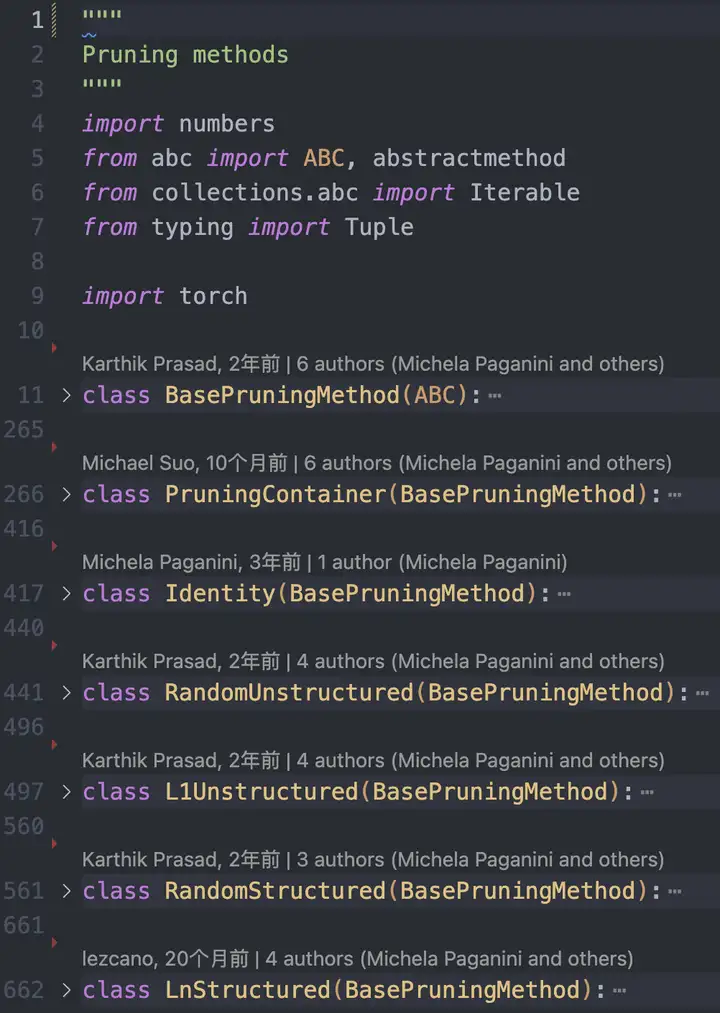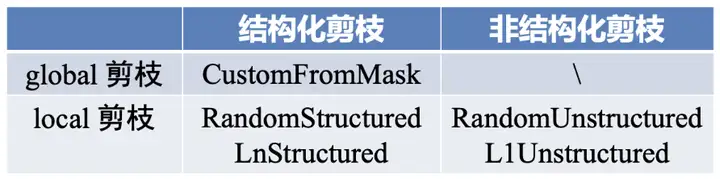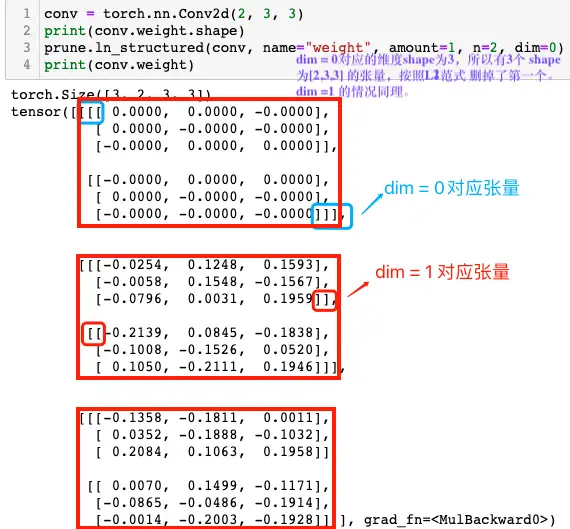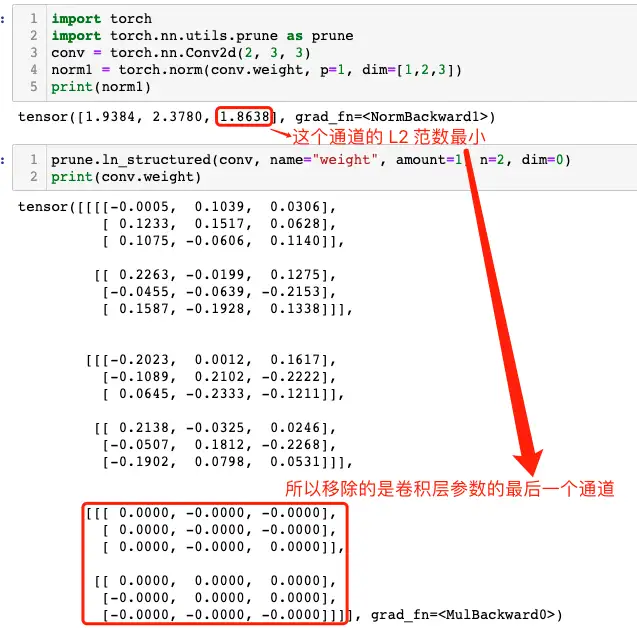# 實踐Pytorch中的模型剪枝方法

## 二，PyTorch 的剪枝

• Random: 簡單地修剪隨機引數。
• Magnitude: 修剪權重最小的引數（例如它們的 L2 範數）

### 2.1，pytorch 剪枝工作原理Pytorch 將原始引數 <param> 複製到名為 <param>_original 的引數中，並建立一個緩衝區來儲存剪枝掩碼 <param>_mask。同時，其也會建立一個模組級的 forward_pre_hook 回撥函式（在模型前向傳播之前會被呼叫的回撥函式），將剪枝掩碼應用於原始權重。

pytorch 剪枝的 api 和教程比較混亂，我個人將做了如下表格，希望能將 api 和剪枝方法及分類總結好。pytorch 中進行模型剪枝的工作流程如下：

1. 選擇剪枝方法（或者子類化 BasePruningMethod 實現自己的剪枝方法）。
2. 指定剪枝模組和引數名稱。
3. 設定剪枝方法的引數，比如剪枝比例等。

### 2.2，區域性剪枝

Pytorch 框架中的區域性剪枝有非結構化和結構化剪枝兩種型別，值得注意的是結構化剪枝只支援區域性不支援全域性。

#### 2.2.1，區域性非結構化剪枝

1，區域性非結構化剪枝（Locall Unstructured Pruning）對應函式原型如下：

``def random_unstructured(module, name, amount)``

1）函式功能

2）函式引數定義：

• module (nn.Module): 需要剪枝的網路層/模組，例如 nn.Conv2d() 和 nn.Linear()。
• name(str): 要剪枝的引數名稱，比如 “weight” 或 “bias”。
• amount (int or float): 指定要剪枝的數量，如果是 0~1 之間的小數，則表示剪枝比例；如果是證書，則直接剪去引數的絕對數量。比如amount=0.2 ，表示將隨機選擇 20% 的元素進行剪枝。

3）下面是 random_unstructured 函式的使用示例。

``````import torch
import torch.nn.utils.prune as prune
conv = torch.nn.Conv2d(1, 1, 4)
prune.random_unstructured(conv, name="weight", amount=0.5)
conv.weight
"""
tensor([[[[-0.1703,  0.0000, -0.0000,  0.0690],
[ 0.1411,  0.0000, -0.0000, -0.1031],
[-0.0527,  0.0000,  0.0640,  0.1666],
[ 0.0000, -0.0000, -0.0000,  0.2281]]]], grad_fn=<MulBackward0>)
"""``````

#### 2.2.2，區域性結構化剪枝

``````def random_structured(module, name, amount, dim)
def ln_structured(module, name, amount, n, dim, importance_scores=None)``````

1）函式功能

2）引數定義

n 表示剪枝的範數，dim 表示剪枝的維度。

• dim = 0： 移除一個神經元。
• dim = 1：移除與一個輸入的所有連線。

• dim = 0(Channels) : 通道 channels 剪枝/過濾器 filters 剪枝
• dim = 1（Neurons）: 二維卷積核 kernel 剪枝，即與輸入通道相連線的 kernel

#### 2.2.3，區域性結構化剪枝示例程式碼

``class torch.nn.Conv2d(in_channels, out_channels, kernel_size, stride=1, padding=0, dilation=1, groups=1, bias=True)````````conv = torch.nn.Conv2d(2, 3, 3)
norm1 = torch.norm(conv.weight, p=1, dim=[1,2,3])
print(norm1)
"""
"""
prune.ln_structured(conv, name="weight", amount=1, n=2, dim=0)
print(conv.weight)
"""
tensor([[[[-0.0005,  0.1039,  0.0306],
[ 0.1233,  0.1517,  0.0628],
[ 0.1075, -0.0606,  0.1140]],
[[ 0.2263, -0.0199,  0.1275],
[-0.0455, -0.0639, -0.2153],
[ 0.1587, -0.1928,  0.1338]]],
[[[-0.2023,  0.0012,  0.1617],
[-0.1089,  0.2102, -0.2222],
[ 0.0645, -0.2333, -0.1211]],
[[ 0.2138, -0.0325,  0.0246],
[-0.0507,  0.1812, -0.2268],
[-0.1902,  0.0798,  0.0531]]],
[[[ 0.0000, -0.0000, -0.0000],
[ 0.0000, -0.0000, -0.0000],
[ 0.0000, -0.0000,  0.0000]],
[[ 0.0000,  0.0000,  0.0000],
[-0.0000,  0.0000,  0.0000],
"""``````dim = 1 的情況：

``````conv = torch.nn.Conv2d(2, 3, 3)
norm1 = torch.norm(conv.weight, p=1, dim=[0, 2,3])
print(norm1)
"""
"""
prune.ln_structured(conv, name="weight", amount=1, n=2, dim=1)
print(conv.weight)
"""
tensor([[[[ 0.0000, -0.0000, -0.0000],
[-0.0000,  0.0000,  0.0000],
[-0.0000,  0.0000, -0.0000]],
[[-0.2140,  0.1038,  0.1660],
[ 0.1265, -0.1650, -0.2183],
[-0.0680,  0.2280,  0.2128]]],
[[[-0.0000,  0.0000,  0.0000],
[ 0.0000,  0.0000, -0.0000],
[-0.0000, -0.0000, -0.0000]],
[[-0.2087,  0.1275,  0.0228],
[-0.1888, -0.1345,  0.1826],
[-0.2312, -0.1456, -0.1085]]],
[[[-0.0000,  0.0000,  0.0000],
[ 0.0000, -0.0000,  0.0000],
[ 0.0000, -0.0000,  0.0000]],
[[-0.0891,  0.0946, -0.1724],
[-0.2068,  0.0823,  0.0272],
"""``````

### 2.3，全域性非結構化剪枝

``````# v1.4.0 版本
def global_unstructured(parameters, pruning_method, **kwargs)
# v2.0.0-rc2版本
def global_unstructured(parameters, pruning_method, importance_scores=None, **kwargs):``````

1）函式功能

2）引數定義

• parameters（(Iterable of (module, name) tuples)）: 修剪模型的引數列表，列表中的元素是 (module, name)。
• pruning_method（function）: 目前好像官方只支援 pruning_method=prune.L1Unstuctured，另外也可以是自己實現的非結構化剪枝方法函式。
• importance_scores: 表示每個引數的重要性得分，如果為 None，則使用預設得分。
• **kwargs: 表示傳遞給特定剪枝方法的額外引數。比如 amount 指定要剪枝的數量。

3）global_unstructured 函式的示例程式碼如下所示。

``````device = torch.device("cuda" if torch.cuda.is_available() else "cpu")
class LeNet(nn.Module):
def __init__(self):
super(LeNet, self).__init__()
# 1 input image channel, 6 output channels, 3x3 square conv kernel
self.conv1 = nn.Conv2d(1, 6, 3)
self.conv2 = nn.Conv2d(6, 16, 3)
self.fc1 = nn.Linear(16 * 5 * 5, 120) # 5x5 image dimension
self.fc2 = nn.Linear(120, 84)
self.fc3 = nn.Linear(84, 10)
def forward(self, x):
x = F.max_pool2d(F.relu(self.conv1(x)), (2, 2))
x = F.max_pool2d(F.relu(self.conv2(x)), 2)
x = x.view(-1, int(x.nelement() / x.shape))
x = F.relu(self.fc1(x))
x = F.relu(self.fc2(x))
x = self.fc3(x)
return x
model = LeNet().to(device=device)
model = LeNet()
parameters_to_prune = (
(model.conv1, 'weight'),
(model.conv2, 'weight'),
(model.fc1, 'weight'),
(model.fc2, 'weight'),
(model.fc3, 'weight'),
)
prune.global_unstructured(
parameters_to_prune,
pruning_method=prune.L1Unstructured,
amount=0.2,
)
# 計算卷積層和整個模型的稀疏度
# 其實呼叫的是 Tensor.numel 內內函式，返回輸入張量中元素的總數
print(
"Sparsity in conv1.weight: {:.2f}%".format(
100. * float(torch.sum(model.conv1.weight == 0))
/ float(model.conv1.weight.nelement())
)
)
print(
"Global sparsity: {:.2f}%".format(
100. * float(
torch.sum(model.conv1.weight == 0)
+ torch.sum(model.conv2.weight == 0)
+ torch.sum(model.fc1.weight == 0)
+ torch.sum(model.fc2.weight == 0)
+ torch.sum(model.fc3.weight == 0)
)
/ float(
model.conv1.weight.nelement()
+ model.conv2.weight.nelement()
+ model.fc1.weight.nelement()
+ model.fc2.weight.nelement()
+ model.fc3.weight.nelement()
)
)
)
# 程式執行結果
"""
Sparsity in conv1.weight: 3.70%
Global sparsity: 20.00%
"""``````

## 三，總結

1. torch.nn.utils.prune.is_pruned(module): 判斷模組 是否被剪枝。
2. torch.nn.utils.prune.remove(module, name)： 用於將指定模組中指定引數上的剪枝操作移除，從而恢復該引數的原始形狀和數值。

## 參考資料

1. How to Prune Neural Networks with PyTorch
2. PRUNING TUTORIAL
3. PyTorch Pruning

「其他文章」